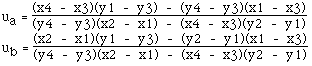# Intersection point of two lines(2 dimensions)

Written by Paul Bourke
April 1989

Sample source code
Contribution by Damian Coventry: example.cpp

This note describes the technique and algorithm for determining the intersection point of two lines (or line segments) in 2 dimensions.The equations of the lines are

Pa = P1 + ua ( P2 - P1 )

Pb = P3 + ub ( P4 - P3 )

Solving for the point where Pa = Pb gives the following two equations in two unknowns (ua and ub)

x1 + ua (x2 - x1) = x3 + ub (x4 - x3)

and
y1 + ua (y2 - y1) = y3 + ub (y4 - y3)

Solving gives the following expressions for ua and ubSubstituting either of these into the corresponding equation for the line gives the intersection point. For example the intersection point (x,y) is

x = x1 + ua (x2 - x1)

y = y1 + ua (y2 - y1)

Notes:

• The denominators for the equations for ua and ub are the same.

• If the denominator for the equations for ua and ub is 0 then the two lines are parallel.

• If the denominator and numerator for the equations for ua and ub are 0 then the two lines are coincident.

• The equations apply to lines, if the intersection of line segments is required then it is only necessary to test if ua and ub lie between 0 and 1. Whichever one lies within that range then the corresponding line segment contains the intersection point. If both lie within the range of 0 to 1 then the intersection point is within both line segments.